﻿ math animation online software ﻿ Fractional Calculus Computer Algebra System, Electrochemistry Software, chat GPT+++=# math animation online software 数学动画

### 2.2.2. plane curve in 2D

Content
1. Classification by plot function 按制图函数分类:
1. plot 制图
2. polar plot 极坐标图
3. plot2D 制图2D
4. Web editor
5. plot3D 立体图
6. spin3D 旋转图3D
7. complex3D 复变函数图3D
8. parametric3D 参数立体图
9. implicit3D 隐函数图3D
2. Classification by appliaction 按应用分类:
3. Classification by Graphics objects
4. Classification by domain
5. Drawing

## 2D animation

1.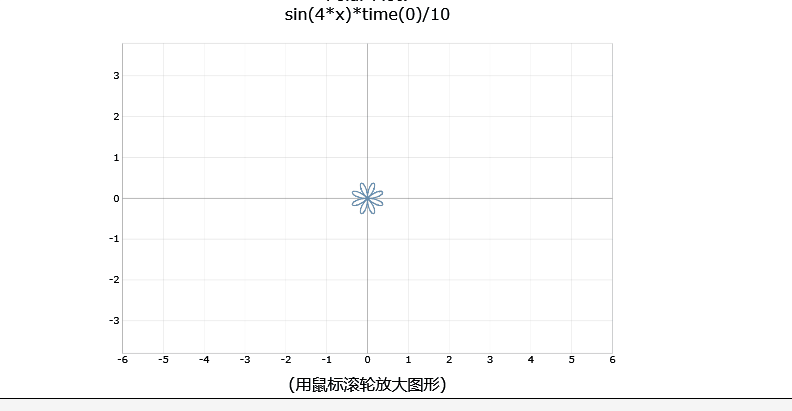flowering by polarplot( sin(4*x)*timer(6) )
2.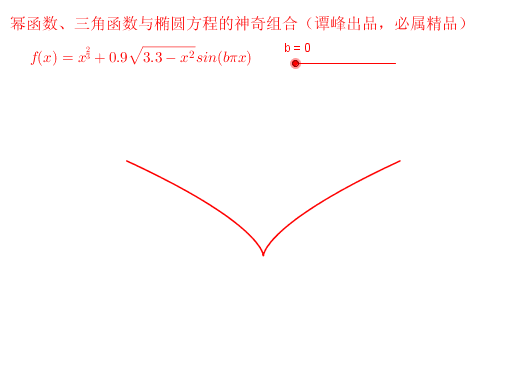I love you by my heart. abs(x)^(2/3)+0.9*sqrt(3.3-x^2)*sin(pi*x*timer(30))
3.A plane curve which is progressively drawn. (Cycloid)
4.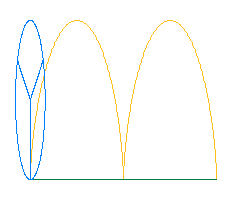The Cycloid is the orbit of a point on a circle rolling along a straight line. Here you can see how this is done precisely, with multiple curve plotting.
5.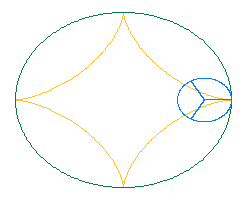By the same principle, the astroid (cos^3(t),sin^3(t)) is the orbit of a point on a circle of radius 1/4 rolling inside a fixed circle of radius 1.
6.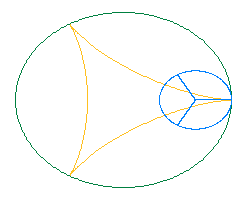We have also the astroid with 3 arcs.
7.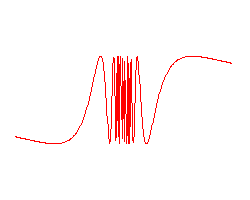Zooming effect on the curve sin(1/x).
8.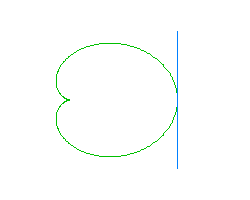A moving tangent along a plane parametric curve (cardioid).
9.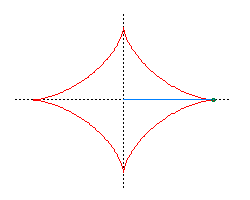The moving tangent along the astroid has a special property: the segment of the tangent cut out by the coordinate axes is of constant length.
10.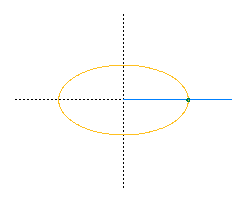How an ellipse is drawn by a point on a segment of fixed length with two extremities on the x and y axes respectively.
11.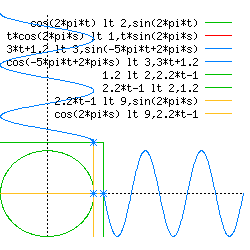An animation which illustrates the functions sin and cos as vertical and horizontal positions of a moving point on a circle.
12.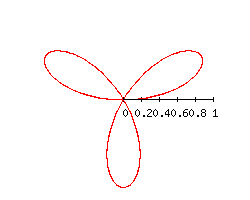Rotating and growing trefoil.

13. Cornu Spiral - the Fresnel cosine and sine integrals.
14. choreographies
15. butterfly
16. square
17. Unwrapping Circle
18. Weierstrass

<= Pre page Next =>

## Reference 参阅

• example 例题
• function graph 制图
• graphics 制图法
• animation 动画 ﻿